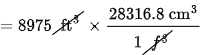×
Get Full Access to Introductory Chemistry - 5 Edition - Chapter 2 - Problem 110p
Get Full Access to Introductory Chemistry - 5 Edition - Chapter 2 - Problem 110p

×

# An iceberg has a volume of 8975 ft3. What is the mass in kilograms of the iceberg? TheISBN: 9780321910295 34

## Solution for problem 110P Chapter 2

Introductory Chemistry | 5th Edition

• Textbook Solutions
• 2901 Step-by-step solutions solved by professors and subject experts
• Get 24/7 help from StudySoup virtual teaching assistantsIntroductory Chemistry | 5th Edition

4 5 1 399 Reviews
16
5
Problem 110P

An iceberg has a volume of $$8975\mathrm{\ ft}^3$$. What is the mass in kilograms of the iceberg? The density of ice is $$0.917\mathrm{\ g}/\mathrm{cm}^3$$.

Equation Transcription:Text Transcription:

8975 ft^

0.917 g/cm^3

Step-by-Step Solution:

Step 1 of 2

Given, Volume of iceberg = 8975.

density of ice = 0.917.

Iceberg mass in kilograms = ?

Mass of Iceberg is :

= Volume of icebergdensity of ice.

Here the volume of iceberg is inand density is in. So,let’s convert the volume into:

1= 28316.8Therefore, 8975inis  :=_____________________

Step 2 of 2

##### ISBN: 9780321910295

The answer to “?An iceberg has a volume of $$8975\mathrm{\ ft}^3$$. What is the mass in kilograms of the iceberg? The density of ice is $$0.917\mathrm{\ g}/\mathrm{cm}^3$$.Equation Transcription:Text Transcription:8975 ft^0.917 g/cm^3” is broken down into a number of easy to follow steps, and 28 words. Since the solution to 110P from 2 chapter was answered, more than 1904 students have viewed the full step-by-step answer. This textbook survival guide was created for the textbook: Introductory Chemistry, edition: 5. The full step-by-step solution to problem: 110P from chapter: 2 was answered by , our top Chemistry solution expert on 05/06/17, 06:45PM. This full solution covers the following key subjects: iceberg, kilograms, Density, ice, mass. This expansive textbook survival guide covers 19 chapters, and 2046 solutions. Introductory Chemistry was written by and is associated to the ISBN: 9780321910295.

## Discover and learn what students are asking

Statistics: Informed Decisions Using Data : Inference about Two Population Standard Deviations
?In Problems 9–14, assume that the populations are normally distributed. Test the given hypothesis. 14. s1 7 s2 at the a = 0.05 level of signi

Unlock Textbook Solution